Next: Worked example 11.6: Oscillating Up: Oscillatory motion Previous: Worked example 11.4: Energy

## Worked example 11.5: Gravity on a new planet

Question: Having landed on a newly discovered planet, an astronaut sets up a simple pendulum of length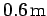, and finds that it makes 51 complete oscillations in 1 minute. The amplitude of the oscillations is small compared to the length of the pendulum. What is the surface gravitational acceleration on the planet?

Answer: The frequency of the oscillations is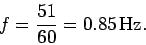Hence, the angular frequency is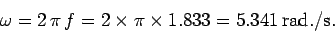Now,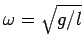for small amplitude oscillations of a simple pendulum. Rearrangement off this formula gives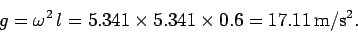Hence, the surface gravitational acceleration is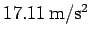.

Richard Fitzpatrick 2006-02-02